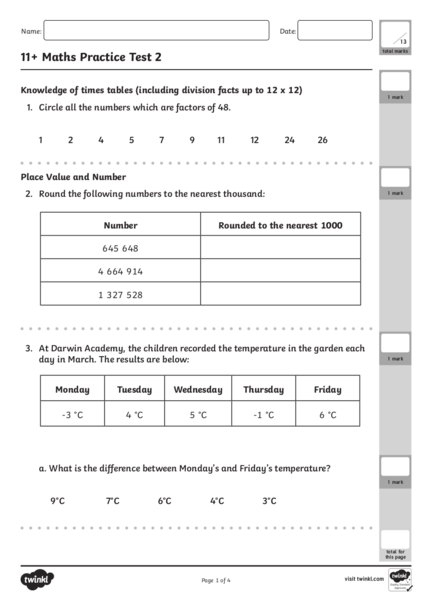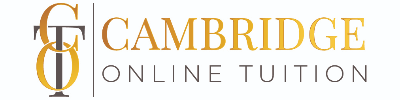# Maths Test for Grade 11Contributed by:Math is all about practice and practice. More you practice, it will become easy for you.
1. Name: Date:
13
11+ Maths Practice Test 2
total marks
Knowledge of times tables (including division facts up to 12 x 12)
1 mark
1. Circle all the numbers which are factors of 48.
1 2 4 5 7 9 11 12 24 26
Place Value and Number
2. Round the following numbers to the nearest thousand: 1 mark
Number Rounded to the nearest 1000
645 648
4 664 914
1 327 528
3. At Darwin Academy, the children recorded the temperature in the garden each
day in March. The results are below: 1 mark
Monday Tuesday Wednesday Thursday Friday
-3 °C 4 °C 5 °C -1 °C 6 °C
a. What is the difference between Monday’s and Friday’s temperature?
1 mark
9°C 7°C 6°C 4°C 3°C
total for
Page 1 of 4
2. 11+ Maths Practice Test 2 2
b. The temperature on Saturday is 5°C lower than Thursday. What is the 1 mark
temperature on Saturday?
4°C 5°C - 4°C - 6°C 6°C
Number: addition, subtraction, multiplication and division
4. Sophie buys a T-shirt for £5.63, a skirt for £7.99 and a pair of shorts. She pays for 1 mark
the items with a £20 note and gets the following coins as change.
How much did the shorts cost? Show your working here.
£
5. How many 7s are there in 387? Round the answer to the nearest whole number.
Show your working here. 1 mark
total for
Page 2 of 4
3. 11+ Maths Practice Test 2 3
6. What is 12.8 x 5.7? Circle the correct answer. 1 mark
a. 70.69 b. 71.08 c. 72.96 d. 73.96 e. 73.69
5
7. What is of 72? Circle the correct answer.
8 1 mark
a. 35 b. 40 c. 45 d. 50 e. 55
Number: fractions (including decimals and percentages)
3
8. Circle the improper fraction that is the same as this mixed number: 5 .
1 mark
7
35 36 37 38 39
a. b. c. d. e.
7 7 7 7 7
9. What is 30 785 ÷ 1000?
1 mark
a. 3.0785 b. 3.785 c. 30.785 d. 307.85 e. 0.3785
total for
Page 3 of 4
4. 11+ Maths Practice Test 2 4
Ratio and Proportion 1 mark
10. You can buy 4 cartons of milk for £2.40. How much will it cost to buy 16 cartons
of milk? Show your working here.
£
11. £3 is shared equally between 5 children. How much will each child receive? 1 mark
a. £1 b. £1.20 c. 60p d. 80p e. 30p
12. A coach left Manchester at 08:52 and arrived in Newquay later that day at
17:23. How long did the journey last?
a. 508 minutes b. 509 minutes
c. 510 minutes d. 511 minutes
**END OF TEST** total for
Page 4 of 4
5. 11+ Maths Practice Test 2 Answers
Knowledge of times tables (including Ratio and Proportion
division facts up to 12 x 12)
10. £9.60 1
1. 1, 2, 4, 12, 24 1
11. c. 60p 1
Place Value and Number
Measurement
646 000
d. 511
2. 4 665 000 1 12. 1
minutes
1 328 000
a. 9°C 1
3. Parent note: For the 11 plus maths
b. -6°C 1 test, it is vital that your child has a
thorough grasp of place value and
Number: addition, subtraction, calculation. They must also have a
multiplication and division sound understanding of all the KS2
maths objectives taken from the DFE
4. £4.12 1 Programme of Study for KS2 maths.
Use this test to help identify any gaps
5. 55 1 in your child’s knowledge of the maths
curriculum. You can then help them by
6. c. 72.96 1 doing some further practise together
in that area. Before each question, it
7. c. 45 1 states which part of the curriculum it is
taken from to help spot where they need
Number: fractions (including decimals further practice.
and percentages)
38
8. d. 1
7
9. c. 30.785 1
Page 1 of 1